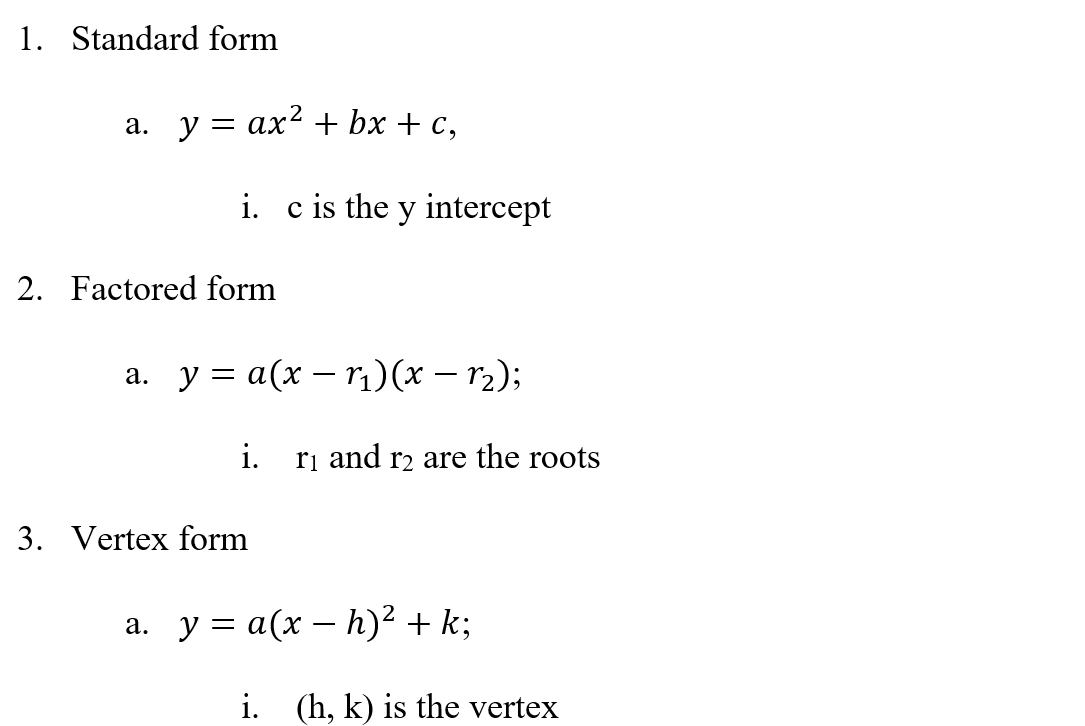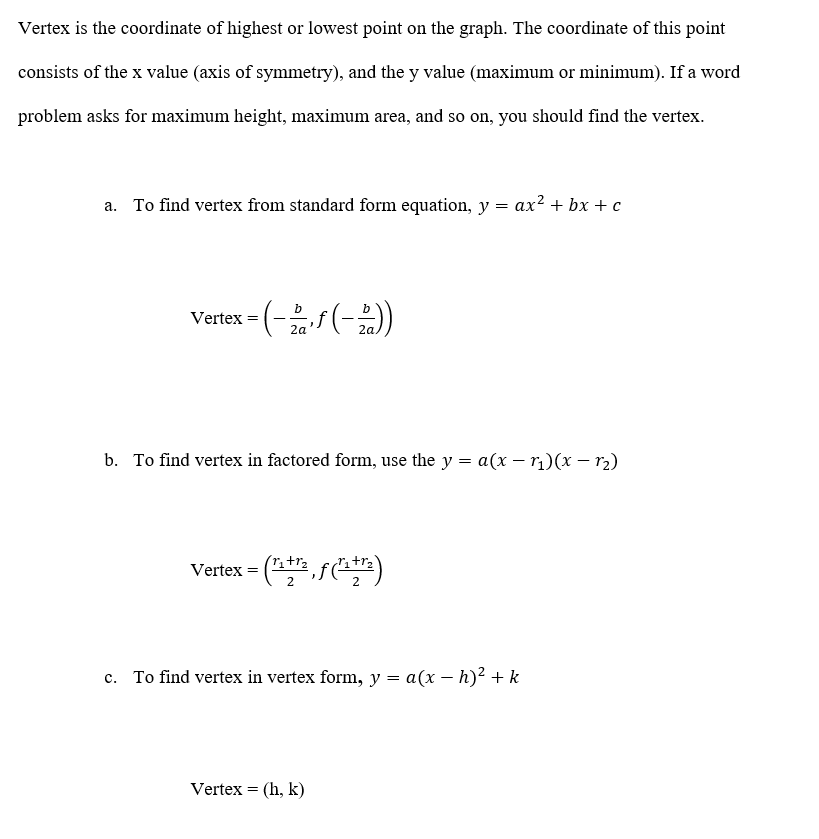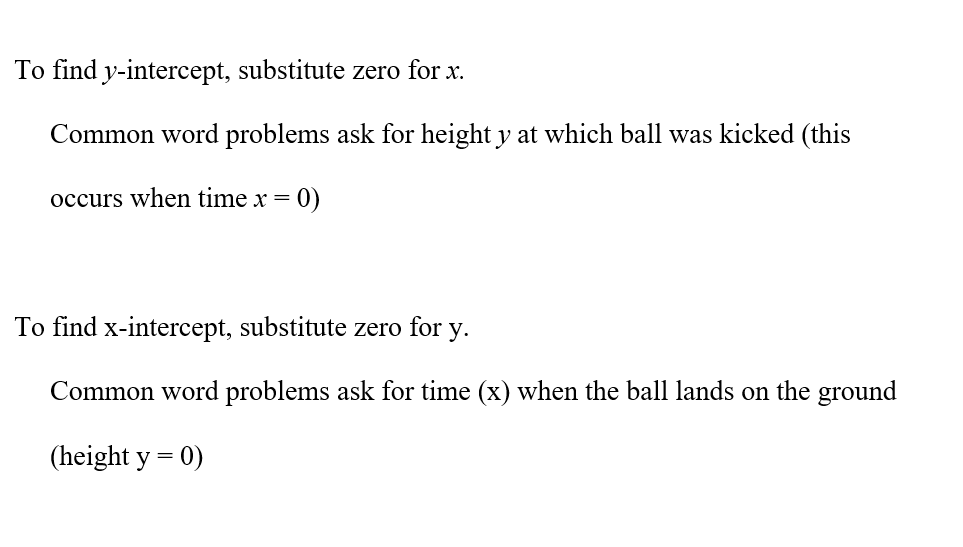Review

What are the three forms of writting the equation of a Quadratic Function?How to find the vertex of a Quadratic function.How to solve a quadratic equation for a variable.How to find the number of solutions that a quadratic equation has.How to find x and y intercepts in a quadratic function.